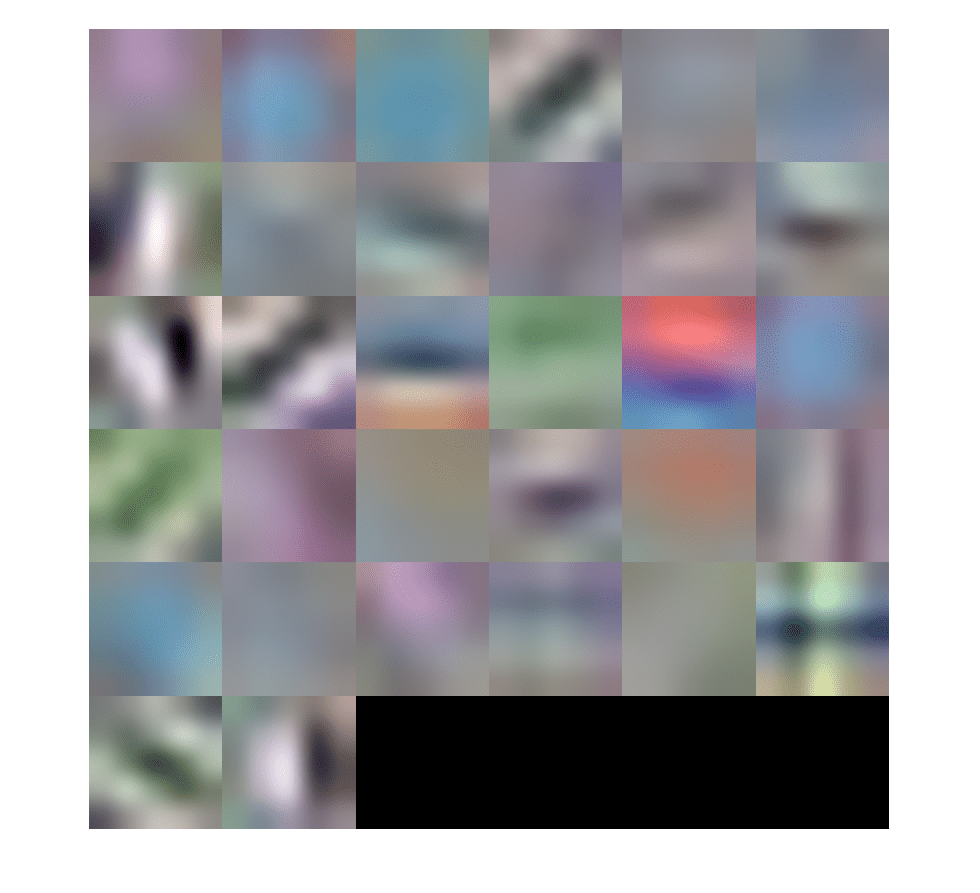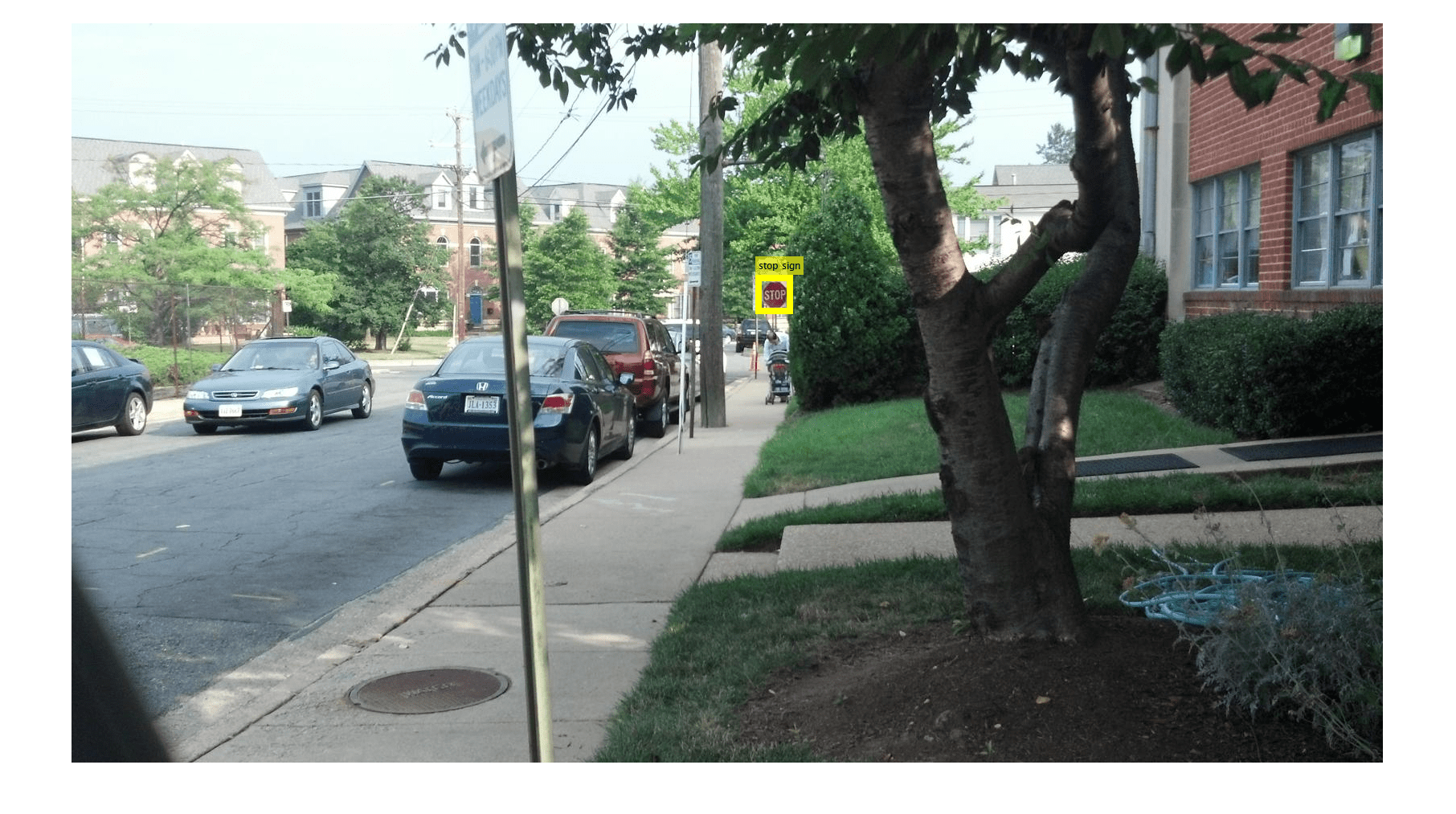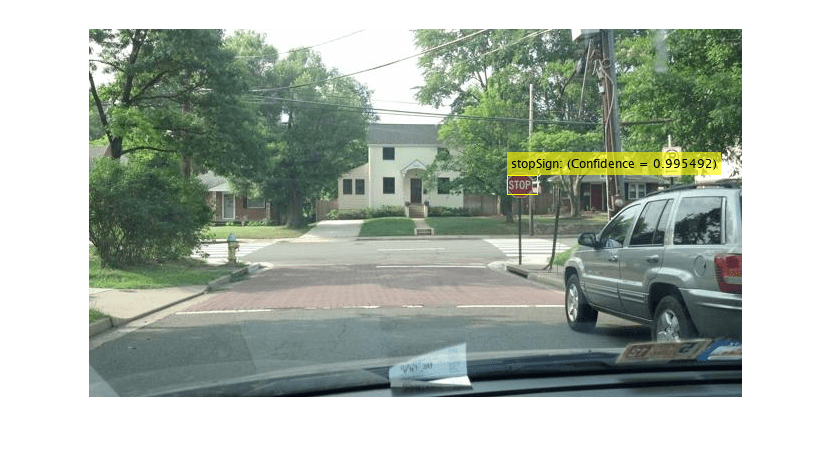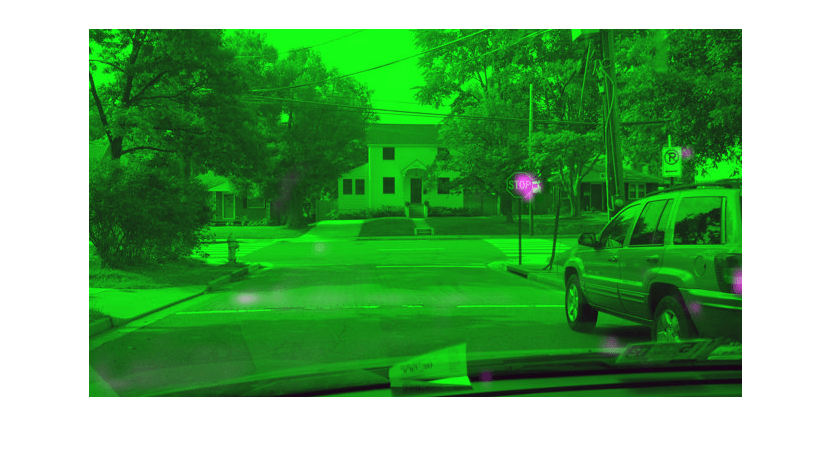# Train Object Detector Using R-CNN Deep Learning

This example shows how to train an object detector using deep learning and R-CNN (Regions with Convolutional Neural Networks).

### Overview

This example shows how to train an R-CNN object detector for detecting stop signs. R-CNN is an object detection framework, which uses a convolutional neural network (CNN) to classify image regions within an image . Instead of classifying every region using a sliding window, the R-CNN detector only processes those regions that are likely to contain an object. This greatly reduces the computational cost incurred when running a CNN.

To illustrate how to train an R-CNN stop sign detector, this example follows the transfer learning workflow that is commonly used in deep learning applications. In transfer learning, a network trained on a large collection of images, such as ImageNet , is used as the starting point to solve a new classification or detection task. The advantage of using this approach is that the pretrained network has already learned a rich set of image features that are applicable to a wide range of images. This learning is transferable to the new task by fine-tuning the network. A network is fine-tuned by making small adjustments to the weights such that the feature representations learned for the original task are slightly adjusted to support the new task.

The advantage of transfer learning is that the number of images required for training and the training time are reduced. To illustrate these advantages, this example trains a stop sign detector using the transfer learning workflow. First a CNN is pretrained using the CIFAR-10 data set, which has 50,000 training images. Then this pretrained CNN is fine-tuned for stop sign detection using just 41 training images. Without pretraining the CNN, training the stop sign detector would require many more images.

Note: This example requires Computer Vision Toolbox™, Image Processing Toolbox™, Deep Learning Toolbox™, and Statistics and Machine Learning Toolbox™.

Using a CUDA-capable NVIDIA™ GPU is highly recommended for running this example. Use of a GPU requires the Parallel Computing Toolbox™. For information about the supported compute capabilities, see GPU Support by Release (Parallel Computing Toolbox).

Download the CIFAR-10 data set . This dataset contains 50,000 training images that will be used to train a CNN.

```cifar10Data = tempdir; url = 'https://www.cs.toronto.edu/~kriz/cifar-10-matlab.tar.gz'; helperCIFAR10Data.download(url,cifar10Data);```

Load the CIFAR-10 training and test data.

`[trainingImages,trainingLabels,testImages,testLabels] = helperCIFAR10Data.load(cifar10Data);`

Each image is a 32x32 RGB image and there are 50,000 training samples.

`size(trainingImages)`
```ans = 1×4 32 32 3 50000 ```

CIFAR-10 has 10 image categories. List the image categories:

```numImageCategories = 10; categories(trainingLabels)```
```ans = 10×1 cell {'airplane' } {'automobile'} {'bird' } {'cat' } {'deer' } {'dog' } {'frog' } {'horse' } {'ship' } {'truck' } ```

You can display a few of the training images using the following code.

```figure thumbnails = trainingImages(:,:,:,1:100); montage(thumbnails) ```

### Create A Convolutional Neural Network (CNN)

A CNN is composed of a series of layers, where each layer defines a specific computation. The Deep Learning Toolbox™ provides functionality to easily design a CNN layer-by-layer. In this example, the following layers are used to create a CNN:

The network defined here is similar to the one described in  and starts with an `imageInputLayer`. The input layer defines the type and size of data the CNN can process. In this example, the CNN is used to process CIFAR-10 images, which are 32x32 RGB images:

```% Create the image input layer for 32x32x3 CIFAR-10 images. [height,width,numChannels, ~] = size(trainingImages); imageSize = [height width numChannels]; inputLayer = imageInputLayer(imageSize)```
```inputLayer = ImageInputLayer with properties: Name: '' InputSize: [32 32 3] Hyperparameters DataAugmentation: 'none' Normalization: 'zerocenter' NormalizationDimension: 'auto' Mean: [] ```

Next, define the middle layers of the network. The middle layers are made up of repeated blocks of convolutional, ReLU (rectified linear units), and pooling layers. These 3 layers form the core building blocks of convolutional neural networks. The convolutional layers define sets of filter weights, which are updated during network training. The ReLU layer adds non-linearity to the network, which allow the network to approximate non-linear functions that map image pixels to the semantic content of the image. The pooling layers downsample data as it flows through the network. In a network with lots of layers, pooling layers should be used sparingly to avoid downsampling the data too early in the network.

```% Convolutional layer parameters filterSize = [5 5]; numFilters = 32; middleLayers = [ % The first convolutional layer has a bank of 32 5x5x3 filters. A % symmetric padding of 2 pixels is added to ensure that image borders % are included in the processing. This is important to avoid % information at the borders being washed away too early in the % network. convolution2dLayer(filterSize,numFilters,'Padding',2) % Note that the third dimension of the filter can be omitted because it % is automatically deduced based on the connectivity of the network. In % this case because this layer follows the image layer, the third % dimension must be 3 to match the number of channels in the input % image. % Next add the ReLU layer: reluLayer() % Follow it with a max pooling layer that has a 3x3 spatial pooling area % and a stride of 2 pixels. This down-samples the data dimensions from % 32x32 to 15x15. maxPooling2dLayer(3,'Stride',2) % Repeat the 3 core layers to complete the middle of the network. convolution2dLayer(filterSize,numFilters,'Padding',2) reluLayer() maxPooling2dLayer(3, 'Stride',2) convolution2dLayer(filterSize,2 * numFilters,'Padding',2) reluLayer() maxPooling2dLayer(3,'Stride',2) ]```
```middleLayers = 9x1 Layer array with layers: 1 '' Convolution 32 5x5 convolutions with stride [1 1] and padding [2 2 2 2] 2 '' ReLU ReLU 3 '' Max Pooling 3x3 max pooling with stride [2 2] and padding [0 0 0 0] 4 '' Convolution 32 5x5 convolutions with stride [1 1] and padding [2 2 2 2] 5 '' ReLU ReLU 6 '' Max Pooling 3x3 max pooling with stride [2 2] and padding [0 0 0 0] 7 '' Convolution 64 5x5 convolutions with stride [1 1] and padding [2 2 2 2] 8 '' ReLU ReLU 9 '' Max Pooling 3x3 max pooling with stride [2 2] and padding [0 0 0 0] ```

A deeper network may be created by repeating these 3 basic layers. However, the number of pooling layers should be reduced to avoid downsampling the data prematurely. Downsampling early in the network discards image information that is useful for learning.

The final layers of a CNN are typically composed of fully connected layers and a softmax loss layer.

```finalLayers = [ % Add a fully connected layer with 64 output neurons. The output size of % this layer will be an array with a length of 64. fullyConnectedLayer(64) % Add an ReLU non-linearity. reluLayer % Add the last fully connected layer. At this point, the network must % produce 10 signals that can be used to measure whether the input image % belongs to one category or another. This measurement is made using the % subsequent loss layers. fullyConnectedLayer(numImageCategories) % Add the softmax loss layer and classification layer. The final layers use % the output of the fully connected layer to compute the categorical % probability distribution over the image classes. During the training % process, all the network weights are tuned to minimize the loss over this % categorical distribution. softmaxLayer classificationLayer ]```
```finalLayers = 5x1 Layer array with layers: 1 '' Fully Connected 64 fully connected layer 2 '' ReLU ReLU 3 '' Fully Connected 10 fully connected layer 4 '' Softmax softmax 5 '' Classification Output crossentropyex ```

Combine the input, middle, and final layers.

```layers = [ inputLayer middleLayers finalLayers ]```
```layers = 15x1 Layer array with layers: 1 '' Image Input 32x32x3 images with 'zerocenter' normalization 2 '' Convolution 32 5x5 convolutions with stride [1 1] and padding [2 2 2 2] 3 '' ReLU ReLU 4 '' Max Pooling 3x3 max pooling with stride [2 2] and padding [0 0 0 0] 5 '' Convolution 32 5x5 convolutions with stride [1 1] and padding [2 2 2 2] 6 '' ReLU ReLU 7 '' Max Pooling 3x3 max pooling with stride [2 2] and padding [0 0 0 0] 8 '' Convolution 64 5x5 convolutions with stride [1 1] and padding [2 2 2 2] 9 '' ReLU ReLU 10 '' Max Pooling 3x3 max pooling with stride [2 2] and padding [0 0 0 0] 11 '' Fully Connected 64 fully connected layer 12 '' ReLU ReLU 13 '' Fully Connected 10 fully connected layer 14 '' Softmax softmax 15 '' Classification Output crossentropyex ```

Initialize the first convolutional layer weights using normally distributed random numbers with standard deviation of 0.0001. This helps improve the convergence of training.

`layers(2).Weights = 0.0001 * randn([filterSize numChannels numFilters]);`

### Train CNN Using CIFAR-10 Data

Now that the network architecture is defined, it can be trained using the CIFAR-10 training data. First, set up the network training algorithm using the `trainingOptions` (Deep Learning Toolbox) function. The network training algorithm uses Stochastic Gradient Descent with Momentum (SGDM) with an initial learning rate of 0.001. During training, the initial learning rate is reduced every 8 epochs (1 epoch is defined as one complete pass through the entire training data set). The training algorithm is run for 40 epochs.

Note that the training algorithm uses a mini-batch size of 128 images. If using a GPU for training, this size may need to be lowered due to memory constraints on the GPU.

```% Set the network training options opts = trainingOptions('sgdm', ... 'Momentum', 0.9, ... 'InitialLearnRate', 0.001, ... 'LearnRateSchedule', 'piecewise', ... 'LearnRateDropFactor', 0.1, ... 'LearnRateDropPeriod', 8, ... 'L2Regularization', 0.004, ... 'MaxEpochs', 40, ... 'MiniBatchSize', 128, ... 'Verbose', true);```

Train the network using the `trainNetwork` (Deep Learning Toolbox) function. This is a computationally intensive process that takes 20-30 minutes to complete. To save time while running this example, a pretrained network is loaded from disk. If you wish to train the network yourself, set the `doTraining` variable shown below to true.

Note that a CUDA-capable NVIDIA™ GPU is highly recommended for training.

```% A trained network is loaded from disk to save time when running the % example. Set this flag to true to train the network. doTraining = false; if doTraining % Train a network. cifar10Net = trainNetwork(trainingImages, trainingLabels, layers, opts); else % Load pre-trained detector for the example. load('rcnnStopSigns.mat','cifar10Net') end```

### Validate CIFAR-10 Network Training

After the network is trained, it should be validated to ensure that training was successful. First, a quick visualization of the first convolutional layer's filter weights can help identify any immediate issues with training.

```% Extract the first convolutional layer weights w = cifar10Net.Layers(2).Weights; % rescale the weights to the range [0, 1] for better visualization w = rescale(w); figure montage(w)```The first layer weights should have some well defined structure. If the weights still look random, then that is an indication that the network may require additional training. In this case, as shown above, the first layer filters have learned edge-like features from the CIFAR-10 training data.

To completely validate the training results, use the CIFAR-10 test data to measure the classification accuracy of the network. A low accuracy score indicates additional training or additional training data is required. The goal of this example is not necessarily to achieve 100% accuracy on the test set, but to sufficiently train a network for use in training an object detector.

```% Run the network on the test set. YTest = classify(cifar10Net, testImages); % Calculate the accuracy. accuracy = sum(YTest == testLabels)/numel(testLabels)```
```accuracy = 0.7456 ```

Further training will improve the accuracy, but that is not necessary for the purpose of training the R-CNN object detector.

Now that the network is working well for the CIFAR-10 classification task, the transfer learning approach can be used to fine-tune the network for stop sign detection.

```% Load the ground truth data data = load('stopSignsAndCars.mat', 'stopSignsAndCars'); stopSignsAndCars = data.stopSignsAndCars; % Update the path to the image files to match the local file system visiondata = fullfile(toolboxdir('vision'),'visiondata'); stopSignsAndCars.imageFilename = fullfile(visiondata, stopSignsAndCars.imageFilename); % Display a summary of the ground truth data summary(stopSignsAndCars)```
```Variables: imageFilename: 41×1 cell array of character vectors stopSign: 41×1 cell carRear: 41×1 cell carFront: 41×1 cell ```

The training data is contained within a table that contains the image filename and ROI labels for stop signs, car fronts, and rears. Each ROI label is a bounding box around objects of interest within an image. For training the stop sign detector, only the stop sign ROI labels are needed. The ROI labels for car front and rear must be removed:

```% Only keep the image file names and the stop sign ROI labels stopSigns = stopSignsAndCars(:, {'imageFilename','stopSign'}); % Display one training image and the ground truth bounding boxes I = imread(stopSigns.imageFilename{1}); I = insertObjectAnnotation(I,'Rectangle',stopSigns.stopSign{1},'stop sign','LineWidth',8); figure imshow(I)```Note that there are only 41 training images within this data set. Training an R-CNN object detector from scratch using only 41 images is not practical and would not produce a reliable stop sign detector. Because the stop sign detector is trained by fine-tuning a network that has been pre-trained on a larger dataset (CIFAR-10 has 50,000 training images), using a much smaller dataset is feasible.

### Train R-CNN Stop Sign Detector

Finally, train the R-CNN object detector using `trainRCNNObjectDetector`. The input to this function is the ground truth table which contains labeled stop sign images, the pre-trained CIFAR-10 network, and the training options. The training function automatically modifies the original CIFAR-10 network, which classified images into 10 categories, into a network that can classify images into 2 classes: stop signs and a generic background class.

During training, the input network weights are fine-tuned using image patches extracted from the ground truth data. The 'PositiveOverlapRange' and 'NegativeOverlapRange' parameters control which image patches are used for training. Positive training samples are those that overlap with the ground truth boxes by 0.5 to 1.0, as measured by the bounding box intersection over union metric. Negative training samples are those that overlap by 0 to 0.3. The best values for these parameters should be chosen by testing the trained detector on a validation set.

For R-CNN training, the use of a parallel pool of MATLAB workers is highly recommended to reduce training time. `trainRCNNObjectDetector` automatically creates and uses a parallel pool based on your parallel preference settings. Ensure that the use of the parallel pool is enabled prior to training.

To save time while running this example, a pretrained network is loaded from disk. If you wish to train the network yourself, set the `doTraining` variable shown below to true.

Note that a CUDA-capable NVIDIA™ GPU is highly recommended for training.

```% A trained detector is loaded from disk to save time when running the % example. Set this flag to true to train the detector. doTraining = false; if doTraining % Set training options options = trainingOptions('sgdm', ... 'MiniBatchSize', 128, ... 'InitialLearnRate', 1e-3, ... 'LearnRateSchedule', 'piecewise', ... 'LearnRateDropFactor', 0.1, ... 'LearnRateDropPeriod', 100, ... 'MaxEpochs', 100, ... 'Verbose', true); % Train an R-CNN object detector. This will take several minutes. rcnn = trainRCNNObjectDetector(stopSigns, cifar10Net, options, ... 'NegativeOverlapRange', [0 0.3], 'PositiveOverlapRange',[0.5 1]) else % Load pre-trained network for the example. load('rcnnStopSigns.mat','rcnn') end```

### Test R-CNN Stop Sign Detector

The R-CNN object detector can now be used to detect stop signs in images. Try it out on a test image:

```% Read test image testImage = imread('stopSignTest.jpg'); % Detect stop signs [bboxes,score,label] = detect(rcnn,testImage,'MiniBatchSize',128)```
```bboxes = 1×4 419 147 31 20 ```
```score = single 0.9955 ```
```label = categorical categorical stopSign ```

The R-CNN object `detect` method returns the object bounding boxes, a detection score, and a class label for each detection. The labels are useful when detecting multiple objects, e.g. stop, yield, or speed limit signs. The scores, which range between 0 and 1, indicate the confidence in the detection and can be used to ignore low scoring detections.

```% Display the detection results [score, idx] = max(score); bbox = bboxes(idx, :); annotation = sprintf('%s: (Confidence = %f)', label(idx), score); outputImage = insertObjectAnnotation(testImage, 'rectangle', bbox, annotation); figure imshow(outputImage)```### Debugging Tips

The network used within the R-CNN detector can also be used to process the entire test image. By directly processing the entire image, which is larger than the network's input size, a 2-D heat-map of classification scores can be generated. This is a useful debugging tool because it helps identify items in the image that are confusing the network, and may help provide insight into improving training.

```% The trained network is stored within the R-CNN detector rcnn.Network```
```ans = SeriesNetwork with properties: Layers: [15×1 nnet.cnn.layer.Layer] ```

Extract the `activations` (Deep Learning Toolbox) from the softmax layer, which is the 14th layer in the network. These are the classification scores produced by the network as it scans the image.

```featureMap = activations(rcnn.Network, testImage, 14); % The softmax activations are stored in a 3-D array. size(featureMap)```
```ans = 1×3 43 78 2 ```

The 3rd dimension in featureMap corresponds to the object classes.

`rcnn.ClassNames`
```ans = 2×1 cell {'stopSign' } {'Background'} ```

The stop sign feature map is stored in the first channel.

`stopSignMap = featureMap(:, :, 1);`

The size of the activations output is smaller than the input image due to the downsampling operations in the network. To generate a nicer visualization, resize `stopSignMap` to the size of the input image. This is a very crude approximation that maps activations to image pixels and should only be used for illustrative purposes.

```% Resize stopSignMap for visualization [height, width, ~] = size(testImage); stopSignMap = imresize(stopSignMap, [height, width]); % Visualize the feature map superimposed on the test image. featureMapOnImage = imfuse(testImage, stopSignMap); figure imshow(featureMapOnImage)```The stop sign in the test image corresponds nicely with the largest peak in the network activations. This helps verify that the CNN used within the R-CNN detector has effectively learned to identify stop signs. Had there been other peaks, this may indicate that the training requires additional negative data to help prevent false positives. If that's the case, then you can increase 'MaxEpochs' in the trainingOptions and re-train.

### Summary

This example showed how to train an R-CNN stop sign object detector using a network trained with CIFAR-10 data. Similar steps may be followed to train other object detectors using deep learning.